• 1、solve函数用法solve('函数方程组')---方程ezplot('函数方程组'，[x1 x2 y1 y2])---画函数的方程root(f,x,k)——f表达式的k阶开根，x是变量。symbolic(象征性的)；polynomial(多项式)；integer(整数)；specify...1、solve函数用法solve('函数方程组')---解方程ezplot('函数方程组'，[x1 x2 y1 y2])---画函数的方程root(f,x,k)——f表达式的k阶开根，x是变量。symbolic(象征性的)；polynomial(多项式)；integer(整数)；specify(指明)；correspond(对应)；constructing(构造)；variable(变量)；short-hand（速记）；notation(符号)；solve（‘方程1’，‘方程2’，‘方程3’，‘方程4’）——可以解方程组2、利用函数对方程展开与合并syms——产生一个变量，如果不产生变量，直接使用expand函数对多项式进行扩展，MATLAB运行会出错，具体用法：2.1 syms x——产生一个变量x;2.2 expand(多项式)——对多项式进行扩展；>> syms y;  >> expand((y-2)*(y+8))  ans =  y^2+6*y-162.3 collect(多项式)——对多项式进行化简>> syms x;  >> collect(x*(x^2 - 2))  ans =  x^3-2*x 2.4 factor（多项式）——对多项式进行因式分解>> syms x;syms y;  >> factor(x^2 - y^2)  ans =   (x-y)*(x+y) 还可以使用一个命令同进对多个方程式进行因式分解：>> factor([x^2-y^2, x^3+y^3])  ans =  [ (x-y)*(x+y), (x+y)*(x^2-x*y+y^2)]2.5 简化simplify 命令>> syms x;  >> simplify((x^4-81)/(x^2-9))  ans = x^2+9 2.6 使用指数和对数函数求解方程lambertw是matlab的一个函数意思是x*e^x=W;W = lambertw(Z) solves W*exp(W) = Z.W = lambertw(K,Z) is the K-th branch of this multi-valued function.不是很清楚lambertw这个表示方式。multi-valued(多值)；2.7 Taylor级数的MATLAB操作函数>> syms x  >> s = taylor(sin(x))  s =  x-1/6*x^3+1/120*x^5 不声明，默认展开到第三项！taylor(f, m)——f是函数，m是展开到多少级。polynomial(多项式)；approximation(近似)；expansion(扩张)；multivariate(多变量)；
展开全文• PAGE / NUMPAGES 用MATLAB解方程的三个实例 作者 周兆安 1对于多项式p(x)=x3-6x2-72x-27求多项式p(x)=0的根可用多项式求根函数rootsp其中p为多项式系数向量即 >p =[1,-6,-72,-27] p = 1.00 -6.00 -72.00 -27.00 p是...
• MATLAB求解方程(1)roots函数功能：用于求解多项式方程的根，以列矢量的形式返回多项式的根。调用格式：r=roots(p)，p是多项式系数按降幂排列所形成的向量。示例：求方程3x2-2x-4=0的根。代码：p=[3,-2,-4];r=roots(p...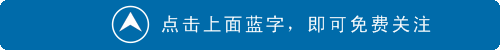MATLAB求解方程(1)roots函数功能：用于求解多项式方程的根，以列矢量的形式返回多项式的根。调用格式：r=roots(p)，p是多项式系数按降幂排列所形成的向量。示例：求方程3x2-2x-4=0的根。代码：p=[3,-2,-4];r=roots(p);方程的根为x1=1.5352，x2=-0.8685.(2)solve函数功能：用于求解方程的符号解。调用格式：r=solve(eq,var),eq为待求解符号函数表达式；var为自变量。[y1,...,yn,parameters,conditions]=solve(eq,vars,Name,value)，eq为待求解符号函数表达式；var为自变量;Name属性主要有'ReturnConditions'，'IgnoreAnalyticConstraints'，'IgnoreProperties'，'MaxDegree'，'PrincipalValue'，'Real';value值有false和true，默认为false。ReturnConditions:是否返回参数条件；ReturnConditions为真时，返回参数条件；value值有false和true，默认为false。IgnoreAnalyticConstraints：方程的化简方法；value值有false和true，默认为false。IgnoreProperties：返回与变量属性不一致的解；value值有false和true，默认为false。MaxDegree：多项式方程的最高阶次；value默认值为2。PrincipalValue：是否返回一个根；PrincipalValue为真时，仅返回一个根，如果方程无根，则返回一个空符号；PrincipalValue为假时，返回所有的根；value值有false和true，默认为false。Real：是否仅返回实根；Real为真时，仅返回实根；value值有false和true，默认为false。示例1：采用solve求解方程sinx=1的解。代码1：syms xeq= sin(x) == 1;r = solve(eqn,x)示例2：采用solve求解方程sin(x) + cos(2*x) = 1的解代码2：syms xeqn = sin(x) + cos(2*x) == 1;solve(eqn, x, 'PrincipalValue', true)solve(eqn, x, 'PrincipalValue', false)PrincipalValue为真时，解为0；PrincipalValue为假时，解为0，π/6，5π/6；示例3：求方程组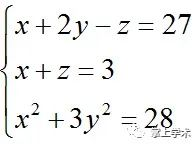的解代码3：[x,y,z]=solve('x+2*y-z=27','x+z=3','x^2+3*y^2=28','x','y','z')注意：待求解变量大于2个时，声明的变量顺序就是solve返回解的顺序。有单引号时，等号用“=”；无单引号时，等号用“==”(3)fsolve函数功能：用最二乘法求非线性方程的根。调用格式：r=fsolve(eq,x0),eq为非线性方程，x0为初始值；用于求非线性方程eq在x0附近的近似解。r=fsolve(eq,x0，options),eq为非线性方程，x0为初始值，options为优化选项，用optimoptions设置选项；用指定的优化选项解方程。示例：求解方程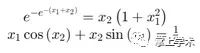的解。代码：fun = @root2d;x0 = [0,0];x = fsolve(fun,x0)单独定义方程为函数root2dfunction F=root2d(x)F(1) = exp(-exp(-(x(1)+x(2)))) - x(2)*(1+x(1)^2);F(2) = x(1)*cos(x(2)) + x(2)*sin(x(1)) - 0.5;求得方程的根为x1=0.3532;x2=0.6061;(4)fzero函数功能：求非线性方程在给定值附近的根调用格式：r=fzero(eq,x0),eq为非线性方程，x0为初始值；用于求非线性方程eq在x0附近的近似解。r=fzero(eq,x0，options),eq为非线性方程，x0为初始值，options为优化选项，用optimoptions设置选项；用指定的优化选项解方程。示例：求解方程sinx=0在3附近的根代码：fun = @sin;x0 = 3; x = fzero(fun,x0)求得方程的根为x=3.1416.(5)linsolve函数功能：求线性方程组的解调用格式：r=linsolve(A,B)，用于求解线性方程组Ax=B的解，B为列向量。r=linsolve(A,B,opts)示例：求线性方程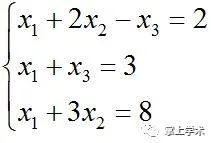的解。代码：A=[1 2 -1; 1 0 1; 1 3 0];B=[2;3;8];X=linsolve(A,B);饭可以一日不吃觉可以一日不睡书不可以一日不读展开全文二分法求方程的根
• 矩阵的基本运算;矩阵的基本运算;矩阵的特征值特征向量特征多项式; 线 性 方 程 组;...例5-21 求非齐次线性方程组的通 MATLAB命令为 B=[1 -1 -1 1 0;1 -1 1 -3 1;1 -1 -2 3 -1/2]; rref(B) ans = 1
• .iuu .iuu .iuu .iuu .iuu .iuu Matlab 多项式运算与代数方程求解器 主要内容 多项式的表达方式 多项式的四则运算 多项式的求导 求多项式的值 求多项式的零点 求线性方程组 求非线性方程的根 符号求解或求根 ....
• 线性代数中最核心的一个操作是不同的矩阵方程，包括线性方程组和特征向量等。在传统计算机上，矩阵方程需要一些精心设计的算法，如高斯消元法、LU分解法，然后在多项式时间内(比如O(N3)，N是矩阵行/列数)获得...线性代数存在于几乎所有的科学和工程领域，比如统计学、物理学、信号处理，和机器学习。线性代数中最核心的一个操作是解不同的矩阵方程，包括解线性方程组和特征向量等。在传统计算机上，解矩阵方程需要一些精心设计的算法，如高斯消元法、LU分解法，然后在多项式时间内(比如O(N3)，N是矩阵行/列数)获得方程解。在大数据时代，人们要处理的数据呈爆发式增长，而很多数据处理本质是一个矩阵方程问题，如谷歌的网页排序(PageRank算法)、利用超级计算机模拟量子效应(解薛定谔方程)。随着摩尔定律的失效，以及由于冯诺依曼架构存储、计算分离的固有限制，想更快、更省地完成数据处理变得越来越难，而计算的时间和能耗效率在大数据时代显得尤为重要。近年来，存内计算(in-memory computing)兴起，通过在存储单元内完成计算将显著提高计算效率。一个典型的存内计算例子是利用阻变存储器(或称忆阻器)的交叉阵列一步完成矩阵-向量乘法的运算，从而快速完成包括训练神经网络在内的大数据任务。然而，一步解矩阵方程，如线性方程组Ax = b，仍然是一个挑战。在我们最新的PNAS文章里，我们报道了利用交叉存储阵列，通过引入反馈电路，可以一步解线性方程组和矩阵的特征向量，进而实现一步解微分方程，包括傅里叶方程、薛定谔方程，和一步完成谷歌的网页排序。对于解线性方程组Ax = b，我们采用的电路如图1(a)所示。电路中包含了一个交叉存储阵列，阵列中每一个行线和列线之间有一个阻变存储器件，它的电导值一一对应于矩阵中的一个元素值。已知向量b由行线的输入电流表示，通过运算放大器(运放)引入的负反馈机制将使行线虚接地，从而列线上运放的输出电压可以表示未知向量x的解值。由于矩阵A以非易失的形式存储在交叉阵列中，一旦施加输入电流，相应的线性方程组就能一步得到解答。实验中，我们存储的矩阵A如图1(a)所示。图1(b)给出了一个相应的线性方程组的实验解，以及它的解析解。可以看到，实验解十分接近解析解(相对误差在3%)，从而证明了我们方案的可行性。逆矩阵也是线性代数中一项非常重要的内容，它也可以通过求解多个线性方程组获得，实验解和解析解的比较如图1(c)所示。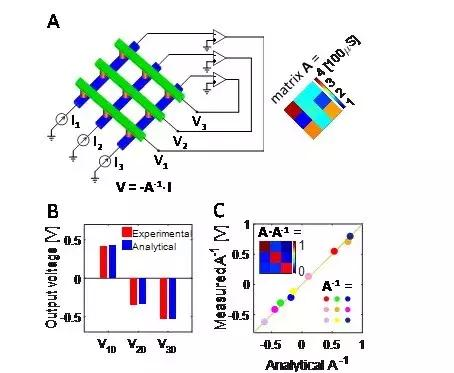图1. 线性方程组求解电路及求解结果。阻变存储器件的电导值只能为正数，因此图1(a)的电路只适用于解系数为正的线性方程组。为了使我们的方案具有普适性，即，解任意实数的线性方程组，我们进一步拓展了电路，如图2(a)所示。图中利用了两个交叉阵列和反相器，实现了两个正矩阵之间的差，从而能够代表任意实数矩阵。基于该普适的线性方程组求解电路，利用有限差分法，我们求解了一个微分方程。如图2(b)所示，在两个电极之间施加一个电压，电极之间导线上的温度分布可以用一维静态傅里叶方程来描述。图2(c)给出了该微分方程的电路解，可以发现与方程的解析解完美重合，从而证明了我们的电路用于快速求解实际问题的可靠性。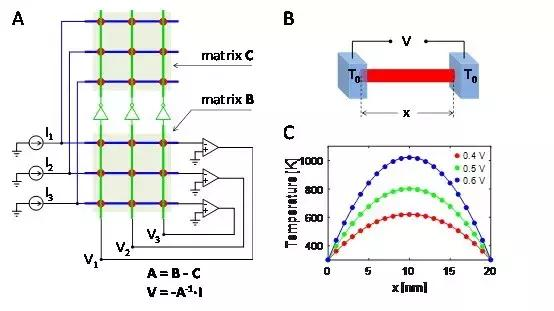图2. 针对任意实数矩阵的线性方程组求解电路，及求解热传导的一维傅里叶方程。基于交叉存储阵列和运放的负反馈机制，我们还能求解矩阵方程Ax = λx，λ为矩阵的特征值，x为相应的特征向量。电路如图3(a)所示，矩阵A依然由交叉阵列表示，λ由跨阻放大器的跨导表示，反相器的输出电压即为待解的x，从而实现一步求解特征向量。需要注意的是，图3(a)电路用于求解正特征值的特征向量，而假如求解负特征值的特征向量，将反相器移去即可，负特征值的绝对值依然由跨阻放大器的跨导表示。和线性方程组求解电路一样，特征向量求解电路也可以拓展到任意实数矩阵。谷歌公司的网页排序算法(PageRank)本质上就是求解一个随机矩阵的最大特征值的特征向量，因此，我们的电路可以用于一步实现网页排序，而不需要繁复的迭代算法，从而为搜索引擎提供加速。图3(b)表示了各科技巨头公司的Wikipedia网页之间的连接情况(网页i指向网页j代表网页i引用网页j)，图3(c)则给出了电路解的排序结果和解析解之间的比较，可以发现二者排序一致。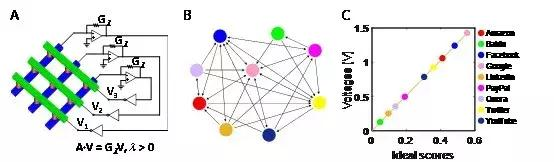图3. 特征向量求解电路及PageRank。薛定谔方程HΨ = EΨ是量子力学的基础，为了获得一个量子系统的波函数分布，必须求解相应的薛定谔方程。作为一个微分方程，薛定谔方程可以通过有限差分法转化为一个特征向量问题。图4(a)是一个一维量子势阱，为了求解它的基态波函数，我们将待求解的波函数离散化，从而将哈密顿量H 转化为一个矩阵，基态能量E 则是最小特征值(负数)，而离散化Ψ则是待求解的特征向量。图4(b)给出了该波函数的电路解，可以看到它很好地描述了一维量子势阱基态的波函数分布，因此证明了我们的电路具有很大的潜力用于加速模拟量子系统，比如在新型材料合成和新型药物开发等领域。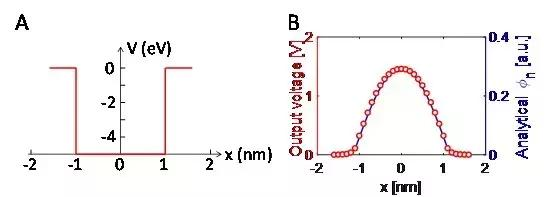图4. 利用特征向量求解电路解一维量子势阱的基态波函数。在我们的工作中，通过利用非易失的阻变存储器(忆阻器)交叉阵列和引入负反馈机制，常见的线性代数问题，包括解线性方程组、解特征向量、解微分方程可以在一步内完成，从而显著提高计算速度，同时降低计算能耗，为未来的存内计算系统应用于实际的大数据问题中打下了坚实基础。该工作由来自意大利米兰理工大学的一支团队完成，团队专注于利用新型存储器件加速传统计算和机器学习的开发。孙仲博士为论文的第一作者，Daniele Ielmini教授为论文的通讯作者。
展开全文• 数学实验Matlab 多项式运算与代数方程求解器主要内容 多项式的表达方式 多项式的四则运算 多项式的求导 求多项式的值 求多项式的零点 求线性方程组 求非线性方程的根 符号求解或求根Matlab 多项式运算 Matlab 中...
• 解方程组二、fsolve() 数值求解：非线性方程三、fzero()：解方程组的根四、roots() 语句的用法:求解多项式的根五、求解线性方程组 一、solve() 语句符号求解 1. 单变量方程 2. 数值方程 >> syms x; &...
文章目录一、solve() 语句符号求解1. 解单变量方程2. 解数值方程3. 解超越方程4. 解方程组二、fsolve() 数值求解：非线性方程三、fzero()：解方程组的根四、roots() 语句的用法:求解多项式的根五、求解线性方程组
一、solve() 语句符号求解
1. 解单变量方程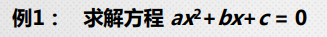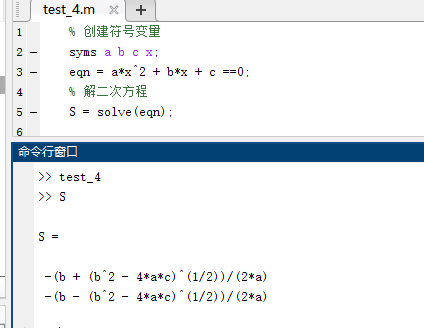2. 解数值方程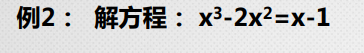>> syms x;
>> eqn= x^3-2*x^2 == x-1;
>> s = solve(eqn,x)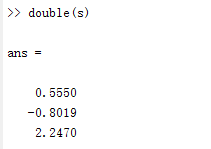3. 解超越方程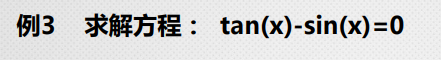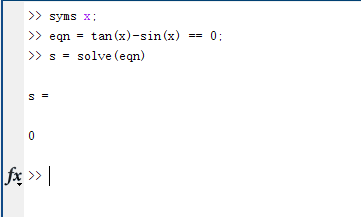并不能得到全部的解
4. 解方程组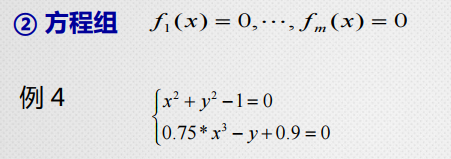clear;
clc;
syms x y;
eqn = [x^2 + y^2 -1 ==0,0.75*x^3 - y + 0.9 ==0];
S = solve(eqn,[x,y]);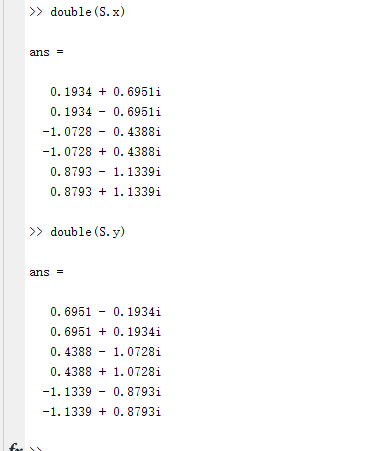二、fsolve() 数值求解：非线性方程
function eq = fun1(x)
eq(1)=2*x(1)-x(2)-exp(-x(1));
eq(2)=-x(1)+2*x(2)-exp(-x(2));
end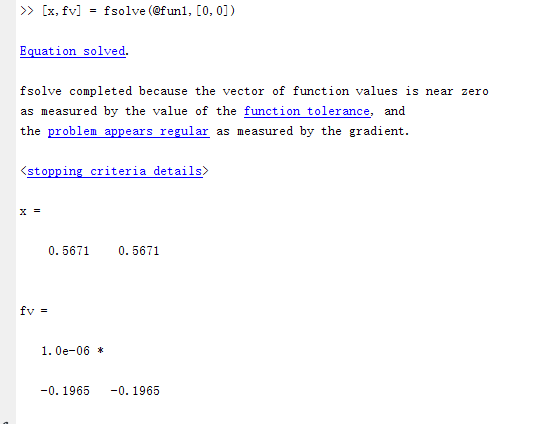三、fzero()：解方程组的根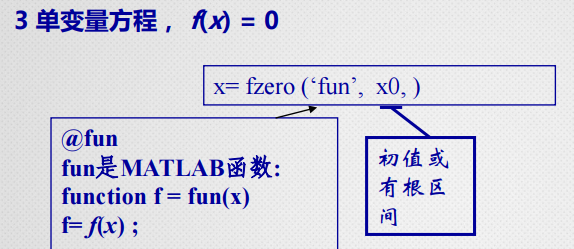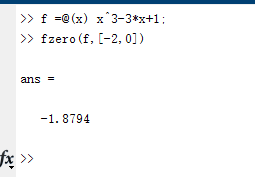四、roots() 语句的用法:求解多项式的根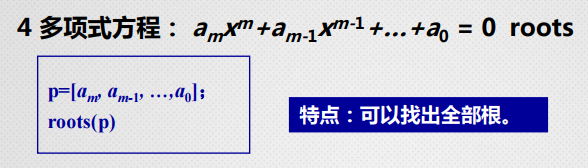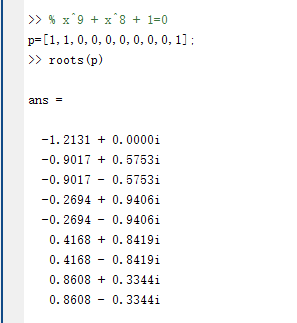五、求解线性方程组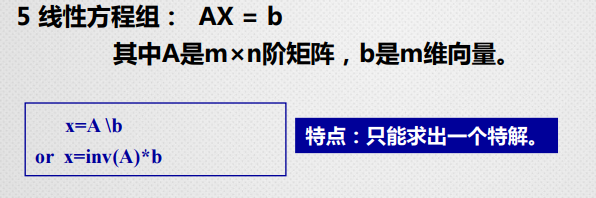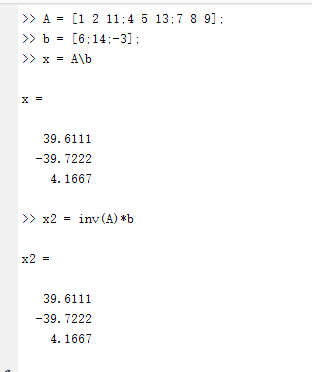展开全文数学建模
• MATLAB 函数 以列向量的形式返回 p 表示的多项式的根。输入 p 是一个包含 n+1 多项式系数的向量，以 xn 系数开头。0系数表示方程中不存在的中间幂。 p=[1,-1,-2]; x=roots(p); 2.solve函数 利用solve函数求方程...
• +线性方程组的直接方法：方程组的逆矩阵解法及其MATLAB程序，三角形方程组的解法及其MATLAB程序，高斯（Gauss）消元法和列主元消元法及其MATLAB程序， LU分解法及其MATLAB程序，误差分析及其两种MATLAB程序，求解...
• 例1：解方程，并且求的导数 fa = [8,0,0,0,0,0,17,0,-3,1];%降幂排列。每项的系数。 xk = roots(fa); disp(xk); % 运行结果： % -0.9578 + 0.5907i % -0.9578 - 0.5907i % -0.0062 + 1.1577i % -0.0062 - 1.1577i ...
• 代码包含了方程求根，多项式的根，一元函数零点，非线性方程组，符号方程解法，牛顿迭代法非线性方程等多种算法的MATLAB实现
• 2．程序具有一定的通用性，程序运行时先输入一个数n表示方程含有的未知数个数，然后输入每个线性方程的系数和常数，求出线性方程组。 二、实验设备和实验环境 安装有C、C++或MATLAB的计算机。 三、算法描述 1．...
• 最小二乘法的基本原理和多项式拟合 转化为系数矩阵是一个对称正定矩阵的方程组 故存在唯一
• MATLAB函数 solve, vpasolve, fsolve, fzero, roots 功能和信息概览 求解函数 多项式型 非多项式型 一维 高维 符号 数值 算法 solve 支持，得到全部符号 若可符号则得到根 支持 支持 ...
• 实用计算方法实验二——多项式最小二乘法拟合 实用计算方法实验二——多项式最小二乘法拟合 采用mlx，即matlab的实时脚本，便于观察...%二次多项式 最小二乘法 矛盾方程组 拟合 %形如a0*1+a1*x+a2*...
• 曲线拟合，即以一条函数曲线去逼近已知测量数据点的过程，考察化学理论值和...式中，bj为拟合系数，N数据点按照上述多项式可组成N个方程，改写成矩阵形式如下： 记作： 由前文知，B的最小二乘为： 则...信号分析 曲线拟合 拟合优度
• Matlab求解线性方程组的命令格式： rank(A)：求矩阵A的秩； rref(A)：求矩阵A的行最简形； null(A)：求系数矩阵为A的齐次方程组的基础系； Null(A,’r’)：求系数矩阵为A的齐次方程组有理数形式的基础系； XA=B...线性代数 数学建模
• 理论知识补充： %矩阵除分为矩阵右除和矩阵左除。 %矩阵右除的运算符号为“/”，设A，B为两个矩阵，则“A/B”是指方程X*B=A的矩阵X。 %矩阵A和B的列数必须是相等。...%求解定解方程组（未知数个数等于方程...
• MATLAB函数 solve, vpasolve, fsolve, fzero, roots 功能和信息概览求解函数多项式型非多项式型一维高维符号数值算法solve支持，得到全部符号若可符号则得到根支持支持支持当无符号时符号方法：利用等式性质...solve函数c语言
• 简介Sympy是一个Python的科学计算库，用一套强大的符号计算体系完成诸如多项式求值、求极限、方程、求积分、微分方程、级数展开、矩阵运算等等计算问题。...解方程组solve()2.求极限limit()3.微分(导数)diff()...
• 其他推导部分和一元一样，本质上还是线性方程组Matlab代码 一元 % 本质上就是n个方程n个未知数，这里的未知数是待求函数的所有系数 % Ac=Y A是由X组成的范德蒙德行列式，根据范德蒙德数据拟合
• 多项式函数（10.2）作为插值函数时，希望通过解方程组（10.3）而得到待定系数 function y=lagrange(x0,y0,x); n=length(x0);m=length(x); for i=1:m z=x(i); s=0.0; for k=1:n p=1.0; for j=1:n if j...
• ## MATLAB常用算法

热门讨论 2010-04-05 10:34:28
DiffParam2 用参数微分法中的中点积分法求非线性方程组的一组 mulFastDown 用最速下降法求非线性方程组的一组 mulGSND 用高斯牛顿法求非线性方程组的一组 mulConj 用共轭梯度法求非线性方程组的一组 mulDamp...
• 展开全部在matlab里面solve命令主要是用来求e5a48de588b63231313335323631343130323136353331333365653331代数方程(即多项式)的,但是也不是说其它方程一个也不能，不过求解非代数方程的能力相当有限，通常只能...
• 第二章 （1）用矩阵除法线性方程组； （2）行列式det、逆inv； （3）特征值、特征向量eig； （4）范数和条件数； 第三章 （1）用roots求多项式的根； （2）用fzero非线性方程； （3）用fsolve非线性方程组...
• DiffParam2 用参数微分法中的中点积分法求非线性方程组的一组 mulFastDown 用最速下降法求非线性方程组的一组 mulGSND 用高斯牛顿法求非线性方程组的一组 mulConj 用共轭梯度法求非线性方程组的一组 mulDamp...
• PAGE / NUMPAGES 有一组关于MH的实验数据附件1.data已知其符合 分布请利用最小二乘法编写程序...b,c的极小点问题 由多元函数取极值的必要条件知a,b,c比为 的即满足 将三元一次线性方程组带入Matlab其程序如下 clear ># matlab解多项式方程组matlab 订阅